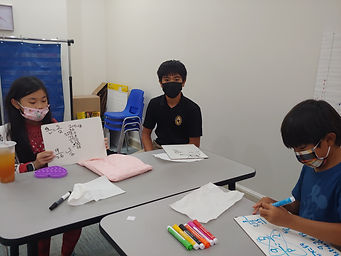## Ms. Mari

### Target 1​

###### Lesson Type:

Review

Data Probability

:

Ratio

Use unit rates to solve problems.

###### 1:

Use unit rates to solve real-world problems.

###### 2:

Calculate and compare unit rates.

###### 3:

Calculate unit rates to compare the cost of two differently prices items.

###### 4:

Use the rules of fractions to solve rates.

6th

###### Vocabulary:

RATIO, RATE, UNIT RATE, PROPORTION

Activities:

- Reviewed solving proportions, some of which containing fractions and decimals.

- Created rates from given quantities in two units and calculated unit rates.

- Calculated and compared unit prices of various items.### Home Exploration

###### Guiding Questions:## Absent Students:

Ryder

### Target 2

:

###### 1:

Use the graphic representation of a variable relationship to determine the proportions present.

6th

###### Vocabulary:

PROPORTIONAL RELATIONSHIP, UNIT RATE

Activities:

Used unit rates to create a table of values and begin to identify proportional relationships.### Home Exploration

###### Guiding Questions:### Target 3

:

###### Vocabulary:

Activities:### Home Exploration